# 5TH ANNIVERSARY AUCTION

### Auction Details

Choose Your Layout >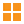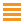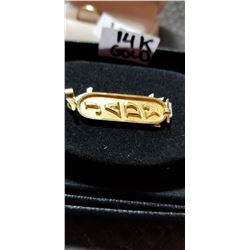81 - Shelf One
CDC Auctions
Shelf One
Bidding Has Concluded

Sold to f***r for (80.00 + 10.40) x 1 = 90.4082 - Shelf One
CDC Auctions
Shelf One
Bidding Has Concluded

Sold to f***r for (50.00 + 6.50) x 1 = 56.50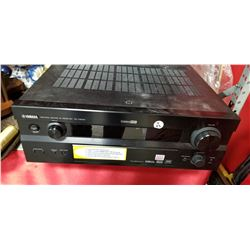83 - Shelf One
CDC Auctions
Shelf One
Bidding Has Concluded

Sold to f***r for (45.00 + 5.85) x 1 = 50.85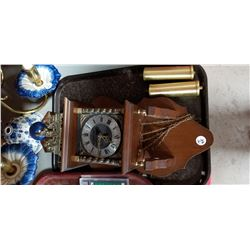85 - Shelf One
CDC Auctions
Shelf One
Bidding Has Concluded

Sold to f***r for (40.00 + 5.20) x 1 = 45.2086 - Shelf One
CDC Auctions
Shelf One
Bidding Has Concluded

Sold to f***r for (5.00 + 0.65) x 1 = 5.6587 - Shelf One
CDC Auctions
Shelf One
Bidding Has Concluded

Sold to f***r for (7.50 + 0.98) x 1 = 8.4888 - Shelf Two
CDC Auctions
Shelf Two
Bidding Has Concluded

Sold to f***r for (60.00 + 7.80) x 1 = 67.80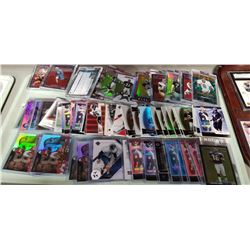89 - Shelf One
CDC Auctions
Shelf One
Bidding Has Concluded

Sold to f***r for (17.50 + 2.28) x 1 = 19.7890 - Shelf One
CDC Auctions
Shelf One
Bidding Has Concluded

Sold to f***r for (70.00 + 9.10) x 1 = 79.10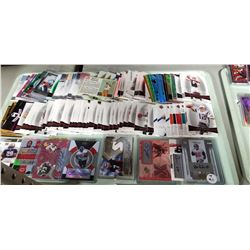91 - Shelf One
CDC Auctions
Shelf One
Bidding Has Concluded

Sold to f***r for (30.00 + 3.90) x 1 = 33.9092 - Shelf One
CDC Auctions
Shelf One
Bidding Has Concluded

Sold to f***r for (40.00 + 5.20) x 1 = 45.2093 - Shelf One
CDC Auctions
Shelf One
Bidding Has Concluded

Sold to f***r for (80.00 + 10.40) x 1 = 90.40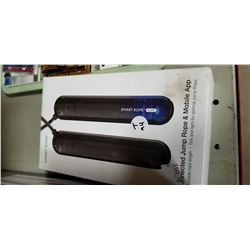94 - Shelf One
CDC Auctions
Shelf One
Bidding Has Concluded

Sold to f***r for (45.00 + 5.85) x 1 = 50.85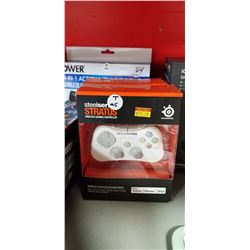95 - Shelf One
CDC Auctions
Shelf One
Bidding Has Concluded

Sold to f***r for (20.00 + 2.60) x 1 = 22.60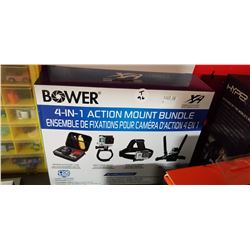96 - Shelf One
CDC Auctions
Shelf One
Bidding Has Concluded

Sold to t*******5 for (20.00 + 2.60) x 1 = 22.6097 - Shelf One
CDC Auctions
Shelf One
Bidding Has Concluded

Sold to f***r for (17.50 + 2.28) x 1 = 19.7898 - Shelf One
CDC Auctions
Shelf One
Bidding Has Concluded

Sold to t*******5 for (15.00 + 1.95) x 1 = 16.95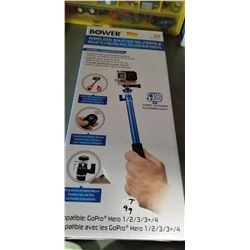99 - Shelf One
CDC Auctions
Shelf One
Bidding Has Concluded

Sold to f***r for (12.50 + 1.63) x 1 = 14.13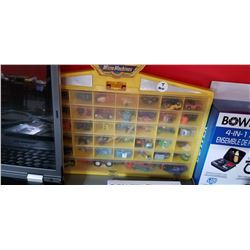100 - Shelf One
CDC Auctions
Shelf One
Bidding Has Concluded

Sold to f***r for (32.50 + 4.23) x 1 = 36.73